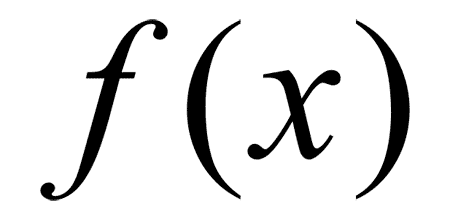# 函数式编程初探(1 + 2) * 3 - 4

var a = 1 + 2;

var b = a * 3;

var c = b - 4;

var result = subtract(multiply(add(1,2), 3), 4);

1. 函数是"第一等公民"

var print = function(i){ console.log(i);};

[1,2,3].forEach(print);

2. 只用"表达式"，不用"语句"

"表达式"（expression）是一个单纯的运算过程，总是有返回值；"语句"（statement）是执行某种操作，没有返回值。函数式编程要求，只使用表达式，不使用语句。也就是说，每一步都是单纯的运算，而且都有返回值。

3. 没有"副作用"

4. 不修改状态

function reverse(string) {

if(string.length == 0) {

return string;

} else {

return reverse(string.substring(1, string.length)) + string.substring(0, 1);

}

}

5. 引用透明

1. 代码简洁，开发快速

Paul Graham在《黑客与画家》一书中写道：同样功能的程序，极端情况下，Lisp代码的长度可能是C代码的二十分之一。

2. 接近自然语言，易于理解

subtract(multiply(add(1,2), 3), 4)

add(1,2).multiply(3).subtract(4)

merge([1,2],[3,4]).sort().search("2")

3. 更方便的代码管理

4. 易于"并发编程"

var s1 = Op1();

var s2 = Op2();

var s3 = concat(s1, s2);

5. 代码的热升级

（完）

## 留言（75条）

「函数式语言的运行速度比较慢」，据说 Haskell 的运行速度与 C++ 相当呢。

cool

http://paulgraham.com/

`引用依云的发言：`

「函数式语言的运行速度比较慢」，据说 Haskell 的运行速度与 C++ 相当呢。

`引用Shiina Luce的发言：`

http://en.wikipedia.org/wiki/Point-free_style

“它属于"结构化编程"的一种，主要思想是把运算过程尽量写成一系列嵌套的函数调用”，阮哥，这是谬误吧……这概念错了啊

`引用一回的发言：`

if(string.length == 0) {
return string;
} else {
return reverse(string.substring(1, string.length)) + string.substring(0, 1);
}

(- ( * ( + 1 2) 3) 4)

http://blog.wolfram.com/2007/07/09/always-the-right-time-for-mathematica/

`引用与非的发言：`

Mathematica和Matlab绝非函数式编程。

Beekka这网站竟然挂了，好多博文配图都没了，好可惜。

“如果程序员每天所写的代码行数基本相同”，这句话成立的前提是“程序员编写代码的速度瓶颈在于打字速度”，这显然是在搞笑，我有理由相信，就算完成相同的功能，C代码量20倍与Lisp，那么使用两种语言开发完成的时间其实是几乎相同的。

lisp主要的问题在于周边库,lib没有,什么都需要自己开发,蛋疼.譬如网络io等...

`引用AnLuoRidge的发言：`

Beekka这网站竟然挂了，好多博文配图都没了，好可惜。

http://erlang-china.org/study/yet-another-pf-guide.html

C++, JAVA, PHP等等这些都引入了Lambda和闭包, 也都是为了方便的实现这个算法.

`引用sokoban的发言：`

Lambda、闭包、惰性求值和Currying真的不算什么函数式编程的“高级特性”，倒不如说这些才是函数式编程的精髓所在。这篇文章竟然把本质的东西全部略过不提

function reverse(string) {

if(string.length == 0) {

return string;

} else {

return reverse(string.substring(1, string.length)) + string.substring(0, 1);

}

}

haskell不错的，不见楼主提及呢。静态化的语言不断引入动态特性，FP，而动态语言性能问题迟迟得不到解决，有点尴尬呀。

`引用sokoban的发言：`

----------------------------------

add(1,2).multiply(3).subtract(4)

------------------------------------

http://blog.sina.com.cn/s/blog_ac9074a201017yf7.html

`引用Mead Lai的发言：`

lisp主要的问题在于周边库,lib没有,什么都需要自己开发,蛋疼.譬如网络io等...

Haskell要开始在业界推广了，Simon Peyton-Jones等人成立了FP complete公司推广haskell，而且要出haskell的IDE。

----------------------------------

add(1,2).multiply(3).subtract(4)

------------------------------------

flip (-) 4 ((*3) (1+2))

subtract (multiply (add 1 2) 3) 4

`引用sokoban的发言：`

Mathematica和Matlab绝非函数式编程。

Matlab不是函数式语言，Mathematica可不一样的（至少支持11种编程范式），没了解清楚之前，话不要说那么满
http://zh.wikipedia.org/wiki/%E5%87%BD%E6%95%B8%E7%A8%8B%E5%BC%8F%E8%AA%9E%E8%A8%80

http://www.oschina.net/translate/scala-expressiveness

http://en.wikipedia.org/wiki/List_of_multi-paradigm_programming_languages

`引用sokoban的发言：`

Mathematica和Matlab绝非函数式编程。

Mathematica不是纯函数式语言（haskell那种），但确实是函数式语言。Mathematica对函数式编程的支持是主流的数学软件中最好的。

subtract(multiply(add(1,2), 3), 4)

add(1,2).multiply(3).subtract(4)

PS：不污染window对象，不用function的prototype进行扩展。

`引用sokoban的发言：`

Mathematica和Matlab绝非函数式编程。

Matlab当然不是,但是Mathematica还是强调函数式编程的,看那些精短的例子就知道了,很多问题可以一句话解决,就是一直套用函数,但是有时会出现性能问题,不过节省人的时间.

`引用levi的发言：`

Java也支持函数式编程，比如：
StringUtils.capitalize(StringUtils.lowerCase(StringUtils.trim("abc")));

merge([1,2],[3,4]).sort().search("2")这个是什么意思？没看懂

`引用stephen的发言：`

“如果程序员每天所写的代码行数基本相同”，这句话成立的前提是“程序员编写代码的速度瓶颈在于打字速度”，这显然是在搞笑，我有理由相信，就算完成相同的功能，C代码量20倍与Lisp，那么使用两种语言开发完成的时间其实是几乎相同的。

`引用climber的发言：`

merge([1,2],[3,4]).sort().search("2")这个是什么意思？没看懂

`引用yansha的发言：`

function reverse(string) {

if(string.length == 0) {

return string;

} else {

return reverse(string.substring(1, string.length)) + string.substring(0, 1);

}

}

`引用a的发言：`

c语言的代码长是因为它没有提供足够的抽象，跟过程不过程没关系。拿matlab跟lisp比比，也没那么夸张吧。

`引用leonac的发言：`

if(string.length == 0) {
return string;
} else {
return reverse(string.substring(1, string.length)) + string.substring(0, 1);
}

“add(1,2).multiply(3).subtract(4)”像这样的连写基本上就是面向对象的功劳，而其中的每个被调用的方法内部实现可以使用函数式编程。

`引用a的发言：`

`引用sokoban的发言：`

`引用xxx的发言：`

haskell不错的，不见楼主提及呢。静态化的语言不断引入动态特性，FP，而动态语言性能问题迟迟得不到解决，有点尴尬呀。

java语言，scala语言，groovy语言都在学习haskell，但，困难在于，对于传统的java，scala，groovy等语言，有大量的用户，如何管理IO，管理状态等副作用，才是根本的需求

`引用skywalker的发言：`

`引用stephen的发言：`

“如果程序员每天所写的代码行数基本相同”，这句话成立的前提是“程序员编写代码的速度瓶颈在于打字速度”，这显然是在搞笑，我有理由相信，就算完成相同的功能，C代码量20倍与Lisp，那么使用两种语言开发完成的时间其实是几乎相同的。

@yansha：

`引用stephen的发言：`

“如果程序员每天所写的代码行数基本相同”，这句话成立的前提是“程序员编写代码的速度瓶颈在于打字速度”，这显然是在搞笑，我有理由相信，就算完成相同的功能，C代码量20倍与Lisp，那么使用两种语言开发完成的时间其实是几乎相同的。

8年了，不知道你现在怎么看。C代码量20倍并不代表你多花20倍时间就能搞定，你会花掉数倍的时间去debug和review，这意味着在你想要达到同等质量的前提下，需要的coder水平完全不同，付出的工资完全不同，对成果质量的预期假设完全不同。

“”“

“”“

«-必填

«-必填，不公开

«-我信任你，不会填写广告链接